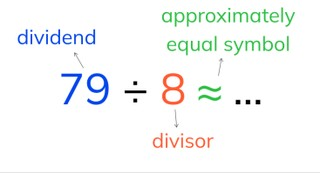Estimated division with numbers to 100

# Estimated division with numbers to 100

Students learn to estimate quotients with numbers to 100.8,000 schools use Gynzy92,000 teachers use Gynzy1,600,000 students use Gynzy

## General

The students learn to estimate the quotient with numbers to 100 by rounding the dividend to a number that is divisible by the divisor.

## Standards

CCSS.Math.Content.3.OA.B.7

## Learning objective

Students will be able to estimate the quotient with numbers to 100.

## Introduction

You practice division table problems with the students in three ways: with visual support, abstract, and in the form of a story problem.

## Instruction

First you explain that the dividend, the divisor, and the approximately equals symbol are. Then you tell the students that with estimated division you always look for a dividend that is divisible by the divisor. For smaller numbers those are numbers from the division table. Tell that estimated division is different than estimated addition or subtraction, in which you can more easily round the numbers to easier numbers. With estimated division you cannot use all rounded numbers. You show estimated division step by step. Explain that you first look at the numbers in the table of the divisor. Find which number is the closest to the dividend in the original problem. Then you can solve the problem. After this the students practice looking for the problem that is the closest to the estimated problem. Next the students are going to estimate division problems on their own. For this, explain that it is sometimes possible to round the dividend to the nearest tens number. Show this step by step using the problem 59 ÷ 3 ≈. After this, have the students estimate the answer to the following problems on their own and ask how they rounded. Then discuss as a group whether the real answers are more or less than the estimate and how you know that. After that, discuss the steps of solving estimated division in a story problem.

Check whether the students can estimate quotients by asking them the following questions:
- When is it useful to estimate a quotient?
- Can you round all problems to a tens number? When is this possible and when is it not possible?

## Quiz

The students test their understanding of estimated division with numbers to 100 through ten exercises. For some of the exercises the students must choose the closest approximate division problem, and for others they must estimate the quotient. Other exercises are story problems.

## Closing

You discuss once again with the students that it is important to be able to estimate quotients, because then you quickly know approximately how much you can divide a number by. Check if the students have understood the instruction section by having them divide a number of glue sticks between three boxes. Approximately how many per box? Are there glue sticks left over?

## Teaching tips

When students have difficulty with estimated division with numbers to 100, then you can have them practice using concrete materials. Begin with smaller division problems, for example 19 blocks that must be divided into 2 groups and then make the problems larger. Have them write out the numbers from the division table to choose the correct number to estimate the problem with.

### The online teaching platform for interactive whiteboards and displays in schools

• Save time building lessons

• Manage the classroom more efficiently

• Increase student engagement